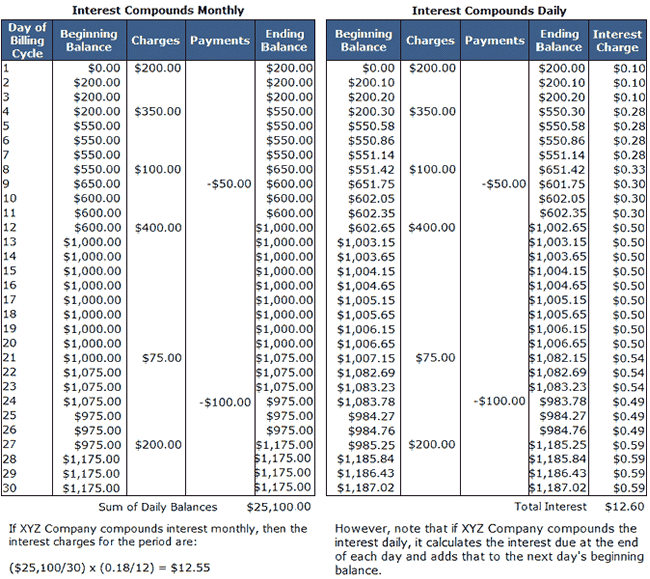## What is Average Daily Balance Method?

The average daily balance method is a way of calculating interest by considering the balance owed or invested at the end of each day of the period rather than the balance owed or invested at the end of the week, month or year.

## How Does Average Daily Balance Method Work?

The frequency of interest compounding affects how lenders and borrowers use the average daily balance method. If interest compounds monthly, then borrowers and lenders use the following formula to calculate interest under the average daily balance method:

(A / D) x (I / P)

Where:
A = the sum of the daily balances in the billing period
D = number of days in the billing period
I = annual interest rate
P = number of billing periods per year (usually 12)

If interest compounds daily, then lenders and borrowers calculate the interest on each day's ending balance and add this interest to the next day's beginning balance. note that ending daily balances reflect the day's charges, payments, deposits, and withdrawals in both versions of this method.

Let's assume you have an XYZ Company credit card that uses the average daily balance method and charges an 18% annual rate of interest. Below is the transaction history for the most recent billing period.Notice that daily compounding generates more interest and leaves the borrower with a higher balance at the end of the billing period. The difference is more pronounced the higher the balance owed. Also notice payments lower the outstanding balance immediately in both cases, which lowers the interest accrued on the remaining balance.

The average daily balance method doesn't just apply to debt; investors are well served to understand how an institution's choice of interest-calculation methods affect the amount of interest deposited into the investor's account.

## Why Does Average Daily Balance Method Matter?

The average daily balance method is just one way lenders and borrowers can calculate interest (the Truth in Lending Act describes acceptable methods). For example, interest calculated using the average daily balance method is usually lower than interest calculated under the previous balance method, which applies interest to the last period's balance. However, the average daily balance method tends to create higher interest charges than the adjusted balance method, where interest based on the period's ending balance.

Because of the variety of interest calculation methods out there, borrowers should compare lender offers and investors should compare investment offers by carefully reading the disclosure accompanying those offers. Depending on the anticipated activity, the borrower might save money and the investor might make more money by preferring one calculation method to another.